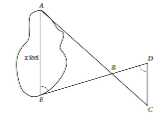# -gre.ge.2 distance by similiar triangles

• MHB
• karush

#### karush

Gold Member
MHB$\textit{not to scale}$
A summer camp counselor wants to find a length, x,
The lengths represented by AB, EB BD,CD on the sketch were determined to be 1800ft, 1400ft, 7000ft, 800 ft respectfully
Segments $AC$ and $DE$ intersect at $B$, and $\angle AEB$ and $\angle CDE$ have the same measure What is the value of $x$?

looks easy but still tricky

$\dfrac{x}{EB}=\dfrac{CD}{BD} =\dfrac{x}{1400}=\dfrac{800}{700}$
multiple thru by 1400 then simplify
$x=\dfrac{800(1400)}{700}=(800)(2)=1600$ hopefully

I thot I posted this problem some time ago here but didn't see the solution in my overall document🕶